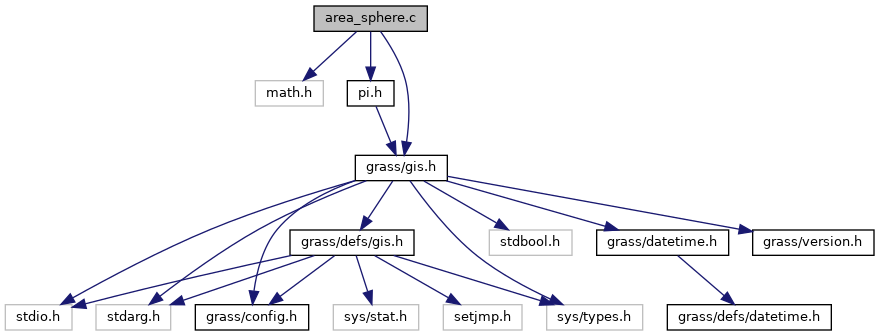GRASS GIS 7 Programmer's Manual  7.9.dev(2021)-e5379bbd7
area_sphere.c File Reference

GIS Library - Sphereical area calculation routines. More...

`#include <math.h>`
`#include <grass/gis.h>`
`#include "pi.h"`
Include dependency graph for area_sphere.c:Go to the source code of this file.

## Functions

void G_begin_zone_area_on_sphere (double r, double s)
Initialize calculations for sphere. More...

double G_darea0_on_sphere (double lat)
Calculates integral for area between two latitudes. More...

double G_area_for_zone_on_sphere (double north, double south)
Calculates area between latitudes. More...

## Detailed Description

GIS Library - Sphereical area calculation routines.

(C) 2001-2009 by the GRASS Development Team

This program is free software under the GNU General Public License (>=v2). Read the file COPYING that comes with GRASS for details.

Definition in file area_sphere.c.

## ◆ G_area_for_zone_on_sphere()

 double G_area_for_zone_on_sphere ( double north, double south )

Calculates area between latitudes.

This routine shows how to calculate area between two lats, but isn't efficient for row by row since G_darea0_on_sphere() will be called twice for the same lat, once as a south then again as a north.

Returns the area between latitudes north and south scaled by the factor s passed to G_begin_zone_area_on_sphere().

Parameters
 north [in] south
Returns
double

Definition at line 70 of file area_sphere.c.

References G_darea0_on_sphere().

## ◆ G_begin_zone_area_on_sphere()

 void G_begin_zone_area_on_sphere ( double r, double s )

Initialize calculations for sphere.

Initializes raster area calculations for a sphere. The radius of the sphere is r and s is a scale factor to allow for calculations of a part of the zone (see G_begin_zone_area_on_ellipsoid()).

Parameters
 r radius of sphere s scale factor

Definition at line 36 of file area_sphere.c.

## ◆ G_darea0_on_sphere()

 double G_darea0_on_sphere ( double lat )

Calculates integral for area between two latitudes.

Parameters
 lat latitude
Returns
area value

Definition at line 48 of file area_sphere.c.

References Radians.

Referenced by G_area_for_zone_on_sphere().